. .
.
Verification of Tafel Equation
.
.

## Objective:

1. Study about the irreversible behaviour of an electrode.
2. Understand the mechanism of electron transfer to an electrode.
3. Determine the current density.
4. Verifying Tafel plot.## Introduction:

Tafel's name is an adjective in the language of all trained electrochemists, yet not too many of them would even know his first name. The fame of the "Tafel law" and "Tafel line" overshadow Tafel's claim to fame as one of the founders of modern electrochemistry.

Until 1893, Tafel had lectured organic chemistry, but after 1893 he lectured physical and general chemistry. By German tradition, this would include lots of electrochemistry, and lots of experimentation. With strychnine reduction, Tafel had truly turned electrochemist. A careful observer, Tafel soon was able to summarize his major and rather far-reaching general deductions from his experimental work.

Professor Julius Tafel, around 1905.
(Courtesy Chemical Institute, Wurzburg University)

## Theory:

### Tafel equation and Tafel plots

Tafel equation governs the irreversible behaviour of an electrode. To understand this we can consider the general mechanism of electron transfer to an electrode.

Consider an electrolyte in which an inert or noble electrode is kept immersed. It is called working electrode, (). Also assume that an oxidised and a reduced species are present near the electrode and exhibit the following electron transfer reaction.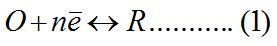is the oxidised andis the reduced species present at equilibrium and is stable in the solution. Let us assume that no other electron transfer reaction other than the above occurs. Let the concentration ofandbeandrespectively and they are very low. An inert electrolyte is also present to minimise IR drop. Along with, a reference electrodeis also kept immersed, to form the cell. Since the potential ofis constant, variation in cell emf is the variation in, and vice versa.

At the thermodynamic equilibrium of the system no net current flows acrossand, no chemical reaction takes place and hence the composition of the solution remains unchanged. The potential of the working electrode will be its equilibrium potential, which according to Nernst equation is,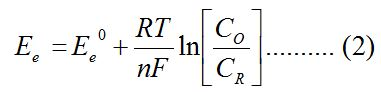Whereis the standard or formal reversible potential and is constant.depends on the ratio of. The square bracketed term should be in terms of activity rather than molar concentration; but at low concentration the replacement is error free.

The equilibrium mentioned above is dynamic. Though no net current flows across the electrodes, both reduction and oxidation takes place at equal rate, so that the composition of the electrolyte does not change. The dynamic flow of electrons or charge in both directions can be written in terms of current densities as follows.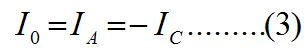Whereis anodic andis cathodic current densities. By convention, anodic current density is given +ve sign and cathodic -ve sign.is known as exchange current density. It may be defined, as "the flow of charge or electrons across an electrochemical system in equilibrium". Its value normally is very low, of the order 10-8 A°. It refers to the extent of both oxidation and reduction that occurs.

The equilibrium situation at an electrode is characterised by equilibrium potential and exchange current density.

For the reaction to have practical significance, a net current should flow and a net reaction either oxidation or reduction should occur. For this the kinetic aspect of the system must be considered. It is to be recalled that thermodynamics fixes the direction and kinetics determines the rate.

For this, let us apply an external potential to, more negative than. This cause an increase in cathodic current and a net quantity ofwill be reduced to. The value of the ratioat the electrode surface will diminish. The magnitude of net cathodic current and the time for the new value oftakes to achieve depend on the rate or the kinetics of the electron transfer reaction. The net cathodic current will be due to the increase in partial cathodic current () and a decrease in partial anodic current () at this new potential. Hence reversible condition changes to irreversible condition. This is achieved by applying a more -ve potential or excess potential than, which is known as over potential. Conversely, it can be argued that ifis made more positive thanby applying external potential more positive than (), a net anodic current will flow through the cell.

To summarize the situation, at the equilibrium potential, no net current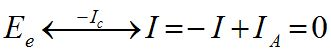Negative to, a net cathodic current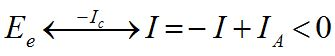Positive to, a net anodic current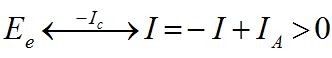The famous Butler-Volmer equation is expressed as: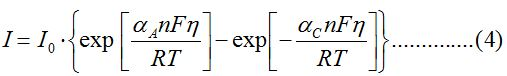From this equation, it can be understand that the measured current density is a function of

(i) over potential ()

(ii) exchange current density () and

(iii) anodic and cathodic transfer coefficients ().

Transfer coefficients are not independent variables. In general,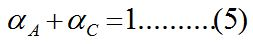For many reaction,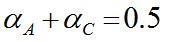Equation (4) indicates that the current density at any over potential is the sum of cathodic and anodic current densities. At the extreme condition of over potential being highly negative, cathodic current density increases while anodic current density becomes negligible. At this stage, the first term in Butler-Volmer equation (4) becomes negligible. The equation can be written as: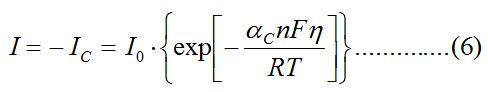When the over potential is higher than above 52 mV, this equation shows that the increase in current is exponential with over potential. The current also depends on. Equation 6 may also be written as: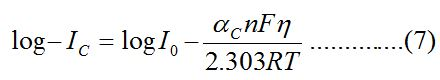Equation 7 is called Cathodic Tafel equation.

Similarly at positive over potentials higher than 52 mV, anodic current density is much higher than cathodic and the cathodic current density  becomes negligible. Hence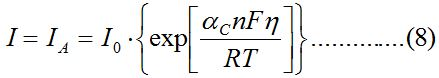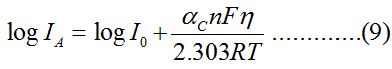Equation (9) is called Anodic Tafel equation.

Whenvalues are plotted against over potential we get Tafel plots. These offer simple method for experimentally determining, transfer coefficients.

### Experimental Determination of I and Tafel Plot

The test electrode is kept immersed in its salt solution. The solution should be very dilute so that the concentration near the surface of the electrode does not differ too much from the bulk concentration. A calomel electrode is kept very close to the test electrode. An inert electrode is also taken which serves as the counter electrode. A DC potential is applied across the test and the counter electrodes, making the test electrode negative. This establishes a potential across the test and the reference electrodes which is read by a very sensitive voltmeter connected in the circuit. From this value the rest potential is subtracted to get the applied potential component on the test electrode. An ammeter connected in series reads the current passing through the circuit. The applied potential is increased which increases the over potential on the cathode (test electrode is made more negative) and the corresponding current value is measured (ammeter reading). In this way the current values are taken for several over potential values making test electrode more and more negative. The log values of these current values are plotted against the over potential on one side.

In the next step the test electrode is connected to the positive terminal and the counter to negative. As done earlier the current is measured for various over potential values and plotted against them on the other side of the graph.

### Significance of Tafel Plots

1. The point of intersection on the Y axis of the extrapolated graph gives the value of I0, the exchange current density, which is otherwise very difficult to determine. It the current passing at equilibrium conditions and a very low value.
2. The transfer coefficients can be determined; from the anodic slope,and from cathodic slopecan be determined. This value is very important in industrial practice. This determines the potential that is to be applied to affect the desired rate of reduction or oxidation.
3. Knowing the value of transfer coefficient for a reaction the number of electrons '' for an unknown reaction can be determined. This reflects on the mechanism of the reaction; that is how many electrons are involved in that step. Whether the reaction is single step or multi step is revealed by this value.
4. The effect ofon current density is shown in the following plot.

1.= 0.25 oxidation is favoured.
2.= 0.50 symmetrical.
3.= 0.75 reduction is favoured.

As cathodic transfer coefficient value increases reduction is favoured and oxidation is not favoured and vice versa for anodic transfer coefficient. The transfer coefficients depend on the pH of the medium; in acidic conditions (low pH) reduction is favoured which is revealed by an increase inCite this Simulator:

.....
..... .....Copyright @ 2020 Under the NME ICT initiative of MHRD